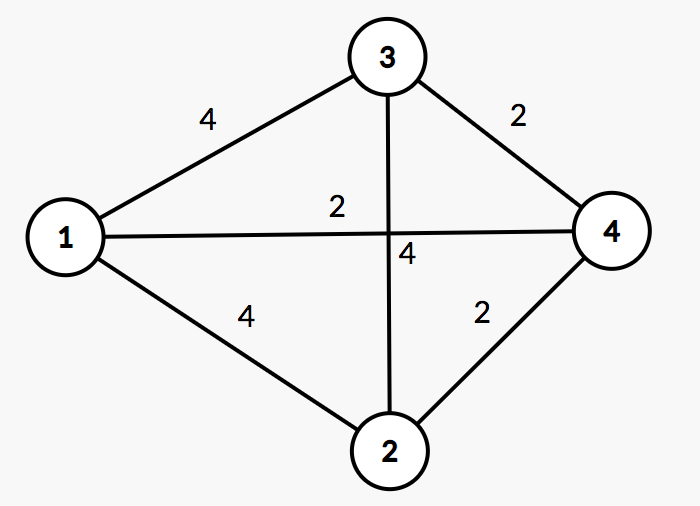# HDU5521 Meeting（建图转化+最短路径）

## Meeting

• Time Limit: 12000/6000 MS (Java/Others)
• Memory Limit: 262144/262144 K (Java/Others)
• Total Submission(s): 5085
• Accepted Submission(s): 1600

### Problem Description

Bessie and her friend Elsie decide to have a meeting. However, after Farmer John decorated his fences they were separated into different blocks. John's farm are divided into n blocks labelled from 1 to n.
Bessie lives in the first block while Elsie lives in the n-th one. They have a map of the farm which shows that it takes they ti minutes to travel from a block in Ei to another block in Ei where Ei (1≤i≤m) is a set of blocks. They want to know how soon they can meet each other and which block should be chosen to have the meeting.

### Input

The first line contains an integer T (1≤T≤6), the number of test cases. Then T test cases follow.

The first line of input contains n and m. $2≤n≤10^5$. The following m lines describe the sets Ei (1≤i≤m). Each line will contain two integers $t_i(1≤t_i≤10^9)$ and Si (Si>0) firstly. Then Si integer follows which are the labels of blocks in Ei. It is guaranteed that $\sum \limits_{i = 1}^{m} {S_i} <= 10^6$.

### Output

For each test case, if they cannot have the meeting, then output "Evil John" (without quotes) in one line.

Otherwise, output two lines. The first line contains an integer, the time it takes for they to meet.

The second line contains the numbers of blocks where they meet. If there are multiple optional blocks, output all of them in ascending order.

### Sample Input

2
5 4
1 3 1 2 3
2 2 3 4
10 2 1 5
3 3 3 4 5
3 1
1 2 1 2

### Sample Output

Case #1: 3
3 4
Case #2: Evil John

### Hint

In the first case, it will take Bessie 1 minute travelling to the 3rd block, and it will take Elsie 3 minutes travelling to the 3rd block. It will take Bessie 3 minutes travelling to the 4th block, and it will take Elsie 3 minutes travelling to the 4th block. In the second case, it is impossible for them to meet.

### Source

2015ACM/ICPC亚洲区沈阳站-重现赛（感谢东北大学）

### 链接

http://acm.hdu.edu.cn/showproblem.php?pid=5521

### 题意

n个点，m个集合，每个集合中任意两点的距离相同（花费时间相同）。现同一时刻，小A从点1出发，小B从点n出发，问他们能否在中间的某些点相遇，按照小A和小B到达这些点的最长时间算，即可能出现小A花1min到达点3，小B花3min到达点3那么时间为max(1min, 3min) = 3min。如果能相遇，输出相遇的最短时间以及在哪些点相遇，从小到大输出。

### 题解#### 代码

#include <bits/stdc++.h>
using namespace std;
typedef long long ll;
const ll inf = 0x3f3f3f3f3f3f3f3f;
const int maxn = 1000010;
ll dist1[maxn], dist2[maxn];
bool vis[maxn];
int n, m;

struct Node
{
int v;
ll c;
Node(int _v, ll _c): v(_v), c(_c) {}
bool operator < (const Node & r) const
{
return c > r.c;
}
};

struct Edge
{
int to, cost;
Edge(int _to, int _cost): to(_to), cost(_cost) {}
};

vector <Edge> edge[maxn];

void addedge(int u, int v, int w)
{
edge[u].push_back(Edge(v, w));
}

void dijkstra(int s, int N, ll dist[]) // 最短路
{
for (int i = 1; i <= N; ++i)
{
dist[i] = inf;
vis[i] = false;
}
dist[s] = 0;
priority_queue <Node> Q;
Q.push(Node(s, dist[s]));
while (!Q.empty())
{
Node tmp = Q.top();
Q.pop();
int u = tmp.v;
if (vis[u])
continue;
vis[u] = true;
for (int i = 0; i < edge[u].size(); ++i)
{
int v = edge[u][i].to, cost = edge[u][i].cost;
if (!vis[v] && dist[v] > dist[u] + cost)
{
dist[v] = dist[u] + cost;
Q.push(Node(v, dist[v]));
}
}
}
}

vector <int> blocks;

int main()
{
int T, t, e, s, kase = 0;
scanf("%d", &T);
while (T--)
{
scanf("%d%d", &n, &m);
for (int i = 1; i <= m; ++i)
{
scanf("%d%d", &t, &e);
for (int j = 1; j <= e; ++j) // 建图，每一个集合外加一个点
{
scanf("%d", &s);
}
}
blocks.clear();
dijkstra(1, n + m, dist1);
dijkstra(n, n + m, dist2);
ll ans = inf, res;
for (int i = 1; i <= n; ++i)
{
res = max(dist1[i], dist2[i]); // 到达该点的最大时间
ans = min(ans, res); // 最短时间去最小值
}
printf("Case #%d: ", ++kase);
if (ans == inf)
printf("Evil John\n");
else
{
printf("%lld\n", ans / 2); // 结果除以2
for (int i = 1; i <= n; ++i)
if (max(dist1[i], dist2[i]) == ans)
blocks.push_back(i);
printf("%d", blocks);
for (int i = 1; i < blocks.size(); ++i)
printf(" %d", blocks[i]);
printf("\n");
}
for (int i = 0; i <= n + m; ++i)
edge[i].clear();
}
return 0;
}

The end.
2018-09-07 星期五